Courses
Courses for Kids
Free study material
Free LIVE classes
More

# NEET Revision Notes for Chemistry Chemical Bonding and Molecular StructureLIVE
Join Vedantu’s FREE Mastercalss

## Chemical Bonding and Molecular Structure Revision Notes for NEET Preparation

The chapter Chemical Bonding and Molecular Structure explains the different properties of various chemical bonds forming between elements. It explains the molecular structure resulting from those bonds and their physical features. All these scientific principles will be discussed elaborately in this chapter to develop a strong conception of chemical bonds in compounds. To make this chapter easy to revise, refer to the Chemical Bonding and Molecular Structure Class 11 notes prepared by the experts at Vedantu.

The experts’ way of explaining the fundamental concepts of this chapter will help students to get a concise understanding of this chapter. The presentation of the principles, definitions, derivations, and formulas of different scientific terms related to chemical bonding will help students prepare this chapter well for NEET.

## NEET Chemistry Revision Notes - Chapter Pages

Last updated date: 27th Sep 2023
Total views: 141k
Views today: 1.40k

## Chemical Bond:

A chemical bond is an attractive force that holds various constituents (atoms, ions, etc.) together in different chemical species together. Energy is released during the formation of a chemical bond.

### Lewis Structures:

• A 'dash' (-) is used to represent a pair of coupled electrons, which is commonly referred to as a 'bond'.

• 'Dots' represent lone pairs or 'non-bonded' electrons.

• Valence electrons are electrons that are found in the outermost shell of an atom.

### Valency

• An element's valency is its ability to form chemical bonds. The combining capacity of an element is defined as its valency.

• The valency of an element determines the number of other atoms that can combine with one atom of that element; for example, carbon has a valency of 4 and hydrogen has a valency of 1. As a result, one carbon atom can combine with four hydrogen atoms to form the methane molecule ${\rm{C}}{{\rm{H}}_{\rm{4}}}$  as follows:Structure of Methane

• The valency of any metal atom is always equal to the number of valence electrons in that atom. For example: sodium (Na) = 2, 8, 1. Therefore, the valency of a sodium atom is 1.

• The valency of the non-metal atom is equal to the difference of 8 and the number of valence electrons in its atom. For example: chlorine (Cl) = 17 = 2 8 7

Therefore, valency of Cl = 8 – 7  = 1

The following are some important elements and their valencies:

1. H, Cl, Br, I, Cu (ous), and so on are monovalent because their valency is one.

2. O, S, Mg, Ca Zn, Fe (ous), Cu (ic), Pb, Co, Ni, and other divalent elements have a valency of two.

3. N, P, Al, Cr, B, and other trivalent elements have a valency of three.

4. C, Si, and other elements are tetravalent as their valency is 4.

Some elements, such as Cu, Fe, and others, have multiple valencies (variable valency). Cu and Hg have + 1 and + 2 valencies, respectively, while Fe has + 2 and + 3 valencies.

## Electronic Theory of Chemical Bonding (Octet Rule)

In 1916, Kossel and Lewis developed the electronic theory of chemical bonding, a theory of chemical combination between atoms based on the inertness of noble gases. "Atoms can combine either by transferring valence electrons from one atom to another (gaining or losing) or by sharing valence electrons in order to have an octet or stable configuration, just like noble gases in their valence shells," according to this theory. This is referred to as the octet rule.

## Exceptions to the Octet Rule:

• Species having an odd number of electrons include the following ${\rm{NO, N}}{{\rm{O}}_{\rm{2}}}{\rm{, N}}{{\rm{O}}_{\rm{3}}}.$

• LiCl, BeH2 and BCl3 make up an incomplete octet for the central atom.

• PF5, SF6 and H2SO4 are the expanded octets for the central atom.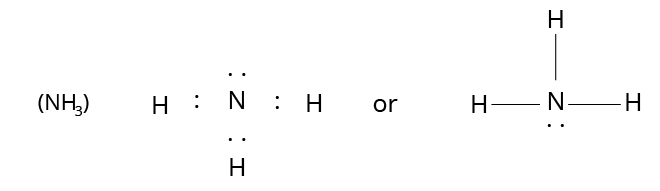Structure of Ammonia

## Formal Charge:

The difference between the number of valence electrons in an isolated atom and the number of electrons assigned to that atom in Lewis structure is referred to as formal charge.

The formula of formal charge can be represented as,

Formal charge = (Total number of valence electron in the free atom) - (Total number of lone pair electrons) - $\dfrac{1}{2}$ (Total number of shared electron i.e. bonding electrons)

### Resonance

• It is impossible to sketch a single Lewis structure for molecules and ions that demonstrate resonance.

• All of the features of such species can be explained by two or more Lewis structures.

The resonance structure of ${{\rm{O}}_{\rm{3}}}$  is shown below.Resonance Structure of Ozone

Here, structures A and B are resonating or canonical structures whereas structure C is the resonance hybrid.

## Types of Chemical Bond

There are three types of chemical bonds:

1. Electrovalent or ionic bond

2. Covalent bond

3. Coordinate bond

## 1. Electrovalent or Ionic Bond

These bonds are formed when one or more electrons are transferred from one atom to another. For example,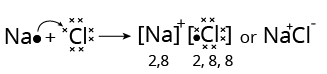Structure of Sodium Chloride

• An electrovalent bond is formed between the majority of electropositive elements, i.e. metals (such as Na, K, Ca, Ba, Mg, etc.) and the majority of electronegative elements, i.e. non-metals (such as O, F, N, S, Cl, Br, etc.).

• The number of electrons lost or taken up by an element is referred to as its electrovalency, which is equal to the charge of a cation or anion, respectively, and these types of compounds are referred to as electrovalent compounds.

• Electropositive elements lose electrons to form cations, while electronegative elements gain electrons to form anions.

• If the difference in electronegativity between the atoms is greater than 1.9, the bond is generally ionic.

### Factors Governing the Formation of Ionic Bonds:

(i) Ionisation Enthalpy (Ionisation Energy)

The amount of energy required to remove an electron from the outermost shell of an isolated atom in the gaseous phase and convert it into a gaseous positive ion is referred to as the ionisation enthalpy of that element.

Obviously, the lower the ionisation enthalpy, the easier it is to remove an electron, i.e., to form a positive ion, and thus the greater the chances of forming an ionic bond. Because the ionisation enthalpy (I.E.) of alkali metals (group I elements) is low, they have a higher tendency to form positive ions.

(ii) Electron Gain Enthalpy (Electron Affinity)

The enthalpy change that occurs when an extra electron is added to an isolated atom in the gaseous phase to form a gaseous negative ion is referred to as an element's electron affinity or electron gain enthalpy. The higher the electron affinity, the more energy is released and the more stable the negative ion produced.

(iii) Lattice Enthalpy (Lattice Energy)

Ionic compounds are formed when positively and negatively charged ions combine to form the compound.

${{\rm{A}}^ + }\left( g \right) + {{\rm{B}}^ - }\left( g \right) \to {{\rm{A}}^ + }{{\rm{B}}^ - }\left( s \right)$

Lattice enthalpy is the energy released when the required number of gaseous positive and negative ions combine to form one mole of the ionic compound.

### Characteristics of Ionic Compounds

The following are the primary characteristics:

1. Ionic compounds are naturally crystalline. Ions are their constituent units. In an ionic lattice, these ions are arranged in a regular pattern; for example, in the NaCl lattice, each sodium ion is bonded with six Cl ions, and each ${\rm{C}}{{\rm{l}}^{\rm{-}}}$  ion is surrounded by six ${\rm{N}}{{\rm{a}}^{\rm{ + }}}$  ions.

2. Because of the strong attraction between oppositely charged ions, ionic compounds are hard and brittle in nature. These solids are low in volatility, stable, and dense.

3. Because of the strong electrostatic force between the ions, these have high melting and boiling points.

4. Because of the mobility of ions, these are good conductors of heat and electricity in a molten state or aqueous medium.

### Variable Electrovalency

Variable electrovalency refers to elements that change their valency and have more than one electrovalency. This is due to the two reasons listed below.

(i) The Effect of Inert Pairs: It has been observed that in the case of heavy elements such as Pb, Bi, and so on, $n{s^2}$  electrons have extra stability and thus do not participate in bond formation. This is known as the inert pair effect. This effect increases as the atomic number in a group increases, for example, Ge and Pb belong to the 14th group of the Periodic Table, but Ge is stable in the ${\rm{G}}{{\rm{e}}^{{\rm{4 + }}}}$  state and Pb is stable in the ${\rm{P}}{{\rm{b}}^{{\rm{2 + }}}}$  state. As a result, ${\rm{P}}{{\rm{b}}^{{\rm{2 + }}}}$  salts are more stable than ${\rm{P}}{{\rm{b}}^{{\rm{4 + }}}}$  salts.

(ii) Unstable Configuration of the Core:

The core of d-block elements has an unstable electron arrangement. As a result, they have variable valency, for example, ${\rm{F}}{{\rm{e}}^{{\rm{2 + }}}}\left( {3{s^2},3{p^6},3{d^6}} \right)$  lose an electron to change into ${\rm{F}}{{\rm{e}}^{{\rm{3 + }}}}$  which cannot lose any more electrons.

## 2. Covalent Bonds

These types of bonds are formed when the atoms in a molecule share an equal number of electrons. Thus, the bond formed by sharing electrons is referred to as a covalent bond, and compounds containing a covalent bond are referred to as covalent compounds.

There are two types of covalent bonds:

(i) Non-Polar Covalent Bonds:

These covalent bonds are formed when two identical atoms or atoms with the same electronegativity come together. The atoms that share electrons have approximately equal electronegativity, and the electron pair is equally shared between them; such a bond is referred to as a polar covalent bond, e.g., ${\rm{C}}{{\rm{l}}_{\rm{2}}}{\rm{, }}{{\rm{O}}_{\rm{2}}}$  and so on.

(ii) Polar Covalent Bonds:

These covalent bonds are formed when different elements or elements with different electronegativity come together. In this bond, the electron pair is attracted to the more electronegative atom, so one atom has a partial negative charge and the other has a partial positive charge, as in HBr, HCl, and so on.

### Characteristics of Covalent Compounds

The following are the primary characteristics:

1. Covalent compounds are typically gases or liquids. Some of the substances are soft solids.

2. These compounds are only slightly soluble in water but extremely soluble in organic solvents such as alcohols, ethers, and so on.

3. Covalent molecules that form hydrogen bonds with water molecules become water-soluble. Lower alcohols, lower carboxylic acids, and so on.

4. They are poor electrical conductors because they lack ions, but when a covalent molecule reacts with water, it dissolves and produces ions, and thus begins to conduct electricity.

### Dipole Moment

• The property that measures the degree of polarity in a bond is known as the dipole moment.

The formula of dipole moment is shown below.

${\rm{Dipole~moment}}\left( \mu \right){\rm{ = Magnitude~of~charges}}\left( e \right) \times {\rm{distance}}\left( d \right)$

• Since diatomic molecules are non-polar, their dipole moments are zero. For example, ${{\rm{H}}_{\rm{2}}}{\rm{, C}}{{\rm{l}}_{\rm{2}}}{\rm{, }}{{\rm{F}}_{\rm{2}}}{\rm{, }}{{\rm{O}}_{\rm{2}}}{\rm{, }}{{\rm{N}}_{\rm{2}}}$

• Because of the large electronegativity differences, the dipole moments of HF, HCl, HBr, and HI are very large.

• Linear molecules with polar covalent bonds, such as ${\rm{C}}{{\rm{O}}_{\rm{2}}}{\rm{, C}}{{\rm{S}}_{\rm{2}}}{\rm{, and~ Be}}{{\rm{F}}_{\rm{2}}}$ . When the bond moments of two bonds are equal but opposite in direction, they cancel each other out, resulting in a dipole moment of zero.

• Because of their symmetrical structure, the dipole moments of ${\rm{C}}{{\rm{H}}_{\rm{4}}}{\rm{, CC}}{{\rm{l}}_{\rm{4}}}{\rm{, and~ B}}{{\rm{F}}_{\rm{3}}}$  are zero.

## 3. Coordinate or Dative Bond

A coordinate covalent bond is one in which both electrons in a shared pair come from the same atom. This type of bond is formed between two atoms, one of which is missing at least two electrons and the other of which has already acquired a stable noble gas configuration.

The atom that donates the electrons is known as the donor, while the atom that accepts the electrons pair is known as the acceptor. An arrow $\left( \to \right)$  pointing from the donor atom to the acceptor atom is commonly used to represent this bond. A hydrogen ion, for example, can form hydronium ion $\left( {{{\rm{H}}_{\rm{3}}}{{\rm{O}}^{\rm{ + }}}} \right)$  by forming a coordinate covalent bond with water molecules.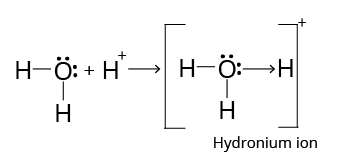Structure of Hydronium Ion

### Coordinate Covalent Bonds in Complex Compounds

Ligands donate a pair of electrons to the central metal atom in complex compounds. Thus, ligands form a coordinate covalent bond in a complex with the central metallic atom. For example: ${\rm{K}}{\left[ {{\rm{Fe}}{{\left( {{\rm{CN}}} \right)}_4}} \right]_{\rm{6}}}$

The central metal atom acts as a Lewis acid in complex salts.

### Characteristics of Coordinate Covalent Compounds

The following are the primary characteristics:

1. Under normal conditions, these exist as solids, liquids, and gases.

2. They are only sparingly soluble in polar solvents such as water, but readily soluble in non-polar solvents.

3. They are poor heat and electricity conductors.

4. They have higher melting and boiling points than purely covalent compounds.

Note:

According to the octet rule, an atom tends to occupy 8 electrons (2 in H like He) in its valence shell, which is a stable arrangement; however, a number of molecules are known to violate the octet rule.

For Example:

(i) ${\rm{Be}}{{\rm{F}}_{\rm{2}}}{\rm{, B}}{{\rm{F}}_{\rm{3}}}$  (incomplete octet)

(ii) ${\rm{NO, N}}{{\rm{O}}_{\rm{2}}}$  (odd number electrons)

(iii)${\rm{PC}}{{\rm{l}}_{\rm{5}}}{\rm{, S}}{{\rm{F}}_{\rm{6}}}$  (expanded octet)

## Valence Shell Electron Pair Repulsion Theory

The Valence Shell theory was the first simple theory proposed to explain the shapes of molecules. The theory of Electron Pair Repulsion (VSEPR). Sidgwick and Powell proposed this theory in 1940, and Nyholm and Gillespie improved on it in 1957. The electron pairs surrounding the central atom repel one another and move so far apart that no further repulsions exist between them. As a result, the molecule has the least amount of energy and the most stability.

Table: Shapes (Geometry) of molecules containing bond pairs only or bond pairs and lone pairs

 Total number of electron pairs Geometry of the Electron Pairs Bond Pairs Lone Pairs Geometry (shape) of the Molecule Illustrative example (other examples) 2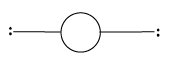Linear 2 0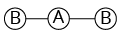Linear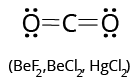${\rm{A}}{{\rm{B}}_{\rm{2}}}$ 3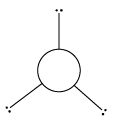Trigonal planar 3 0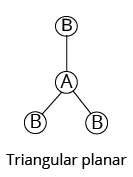Triangular planar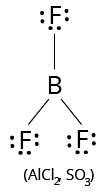${\rm{A}}{{\rm{B}}_{\rm{3}}}$ 2 1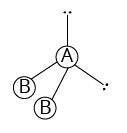Bent (V-shape)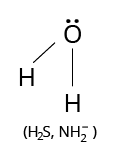${\rm{A}}{{\rm{B}}_{\rm{2}}}{\rm{L}}$Tetrahedral 4 0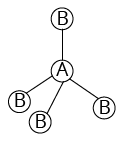Tetrahedral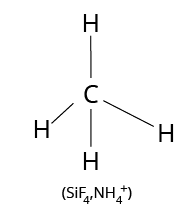${\rm{A}}{{\rm{B}}_{\rm{4}}}$ 3 1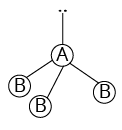Trigonal pyramidal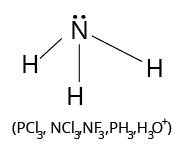${\rm{A}}{{\rm{B}}_{\rm{3}}}{\rm{L}}$ 2 2Bent${\rm{A}}{{\rm{B}}_{\rm{2}}}{{\rm{L}}_{\rm{2}}}$ 5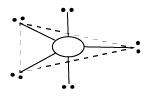Trigonal bipyramidal 5 0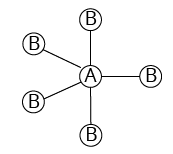Trigonal bipyramidal ${\rm{PC}}{{\rm{l}}_{\rm{5}}}$ ${\rm{A}}{{\rm{B}}_{\rm{5}}}$ 4 1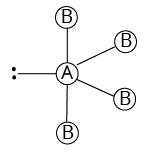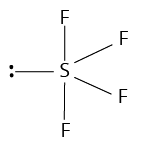${\rm{A}}{{\rm{B}}_{\rm{4}}}{\rm{L}}$ 3 2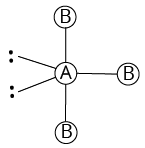T-shaped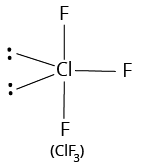${\rm{A}}{{\rm{B}}_{\rm{3}}}{{\rm{L}}_{\rm{2}}}$ 2 3Linear ${\rm{Xe}}{{\rm{F}}_{\rm{2}}}$ ${\rm{A}}{{\rm{B}}_{\rm{2}}}{{\rm{L}}_{\rm{3}}}$ 6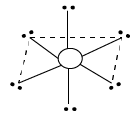6 0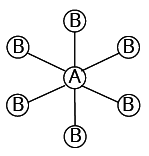Octahedral ${\rm{S}}{{\rm{F}}_{\rm{6}}}$ ${\rm{A}}{{\rm{B}}_{\rm{6}}}$ 5 1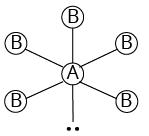Square pyramidal $\begin{array}{l}{\rm{Cl}}{{\rm{F}}_{\rm{6}}}\\\left( {{\rm{I}}{{\rm{F}}_{\rm{5}}}{\rm{, Br}}{{\rm{F}}_{\rm{5}}}} \right)\end{array}$ ${\rm{A}}{{\rm{B}}_{\rm{5}}}{\rm{L}}$ 4 2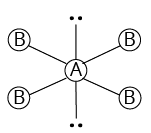Square planar ${\rm{Xe}}{{\rm{F}}_{\rm{4}}}$ ${\rm{A}}{{\rm{B}}_{\rm{4}}}{{\rm{L}}_{\rm{2}}}$

Image: Geometry of molecules

Alt: Shape of molecules containing lone pairs and bond pairs

Calculation of the total number of electron pairs, bond pairs, and lone pairs and predicting the shapes of the molecules and ions.

1. Total no. of electron pairs around the central atom $= \dfrac{1}{2}$  (No. of valence electrons of central atom + No. of atoms linked to central atom by single bonds)

2. The number of bond pairs (shared pairs) is equal to the number of atoms linked to the central atom by single bonds.

3. The number of lone pairs is equal to the difference of the total number of electron pairs and the number of shared pairs.

Example:

On the basis of VSEPR theory, predict the shapes of the following:

$\left( {\rm{i}} \right){\rm{ Cl}}{{\rm{F}}_{\rm{3}}}{\rm{ }}\left( {{\rm{ii}}} \right){\rm{ Br}}{{\rm{F}}_{\rm{5}}}$

(i) Shape of ${\rm{Cl}}{{\rm{F}}_{\rm{3}}}$

The number of valence electrons of the central chlorine atom is 7.

The number of atoms linked to it by single bonds is 3.

Therefore, the total number of electron pairs around chlorine atom $= \dfrac{{7 + 3}}{2} = 5$

The number of bond pairs is equal to number of atoms linked to chlorine $= 3$

Therefore, the number of lone pairs $= 5 - 3 = 2$

Thus, the molecule is of the type ${\rm{A}}{{\rm{B}}_{\rm{3}}}{{\rm{L}}_{\rm{2}}}.$

Hence, it is T-shaped.

(ii) Shape of ${\rm{Br}}{{\rm{F}}_{\rm{5}}}$

The number of valence electrons of the central bromine atom is 7.

The number of atoms linked to it by single bonds is 5.

Therefore, the total number of electron pairs around bromine atom $= \dfrac{{7 + 5}}{2} = 6$

The number of bond pairs is equal to number of atoms linked to Br = 5

Therefore, the number of lone pairs $= 6 - 5 = 1$

Thus, the molecule is of the type ${\rm{A}}{{\rm{B}}_{\rm{5}}}{\rm{L}}.$

Hence, it has a square pyramidal shape.

### Hybridization

Hybridisation is defined as the mixing of atomic orbitals belonging to the same atom but with slightly different energies, resulting in energy redistribution between them and the formation of new orbitals with equal energies and identical shapes. Hybrid orbitals are the new orbitals formed as a result of this process.

### Some Important Hybridisation Points:

1. Only orbitals with roughly equal energies that belong to the same atom or ion can undergo hybridization.

2. The total number of hybrid orbitals produced equals the total number of atomic orbitals mixed.

3. It is not required for all half-filled orbitals to participate in hybridization. Similarly, it is not necessary for hybridization to involve only half-filled orbitals. Even fully filled orbitals with slightly different energies can take part.

## Type of Hybridization

### 1. Diagonal or sp Hybridization

When one s and one p orbital from the same main shell of an atom combine to form two new equivalent orbitals, the process is known as sp hybridization or diagonal hybridization. The newly formed orbitals are known as sp hybrid orbitals.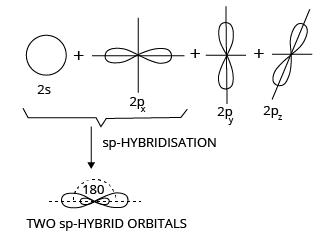SP Hybridization

### 2. sp2 or Trigonal Hybridization

When one s and two p orbitals from the same shell of an atom combine to form three new equivalent orbitals, this is known as sp2 hybridization or trigonal hybridization. The newly formed orbitals are known as sp2 hybrid orbitals.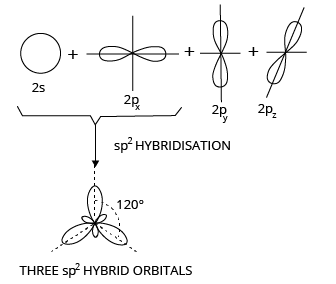sp2 Hybridization

## Hydrogen Bond

The attractive interaction of a hydrogen atom with an electronegative atom from another molecule or chemical group, such as nitrogen (N), oxygen (O), or fluorine (F), is known as a hydrogen bond. It is more powerful than the van der Waals interaction and has an energy range of 5 to ${\rm{30 kJ/mol}}{\rm{.}}$  It can be found in both inorganic molecules like water and organic molecules like DNA.

Hydrogen bonds can be classified into two types.

(i) Intermolecular Hydrogen Bonding:

It occurs between different molecules of a substance, resulting in increased water solubility and a high boiling point.

For example: ${\rm{HF, }}{{\rm{H}}_{\rm{2}}}{\rm{O, }}{{\rm{C}}_{\rm{2}}}{{\rm{H}}_{\rm{5}}}{\rm{OH etc}}{\rm{.}}$

${\rm{H}} - {\rm{F}}......{\rm{H}} - {\rm{F}}......{\rm{H}} - {\rm{F}}$

(ii) Intramolecular Hydrogen Bonding:

It is found in different parts of a single molecule, resulting in decreased water solubility and a low boiling point, as in o-nitrophenol.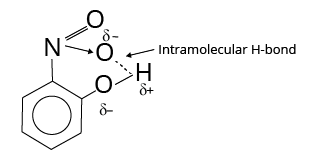Intramolecular Hydrogen Bonding

• Metals have a metallic bond.

• Soft metals, such as Na and K, have a weak metallic bond and can thus be cut with a knife.

• Because of the large number of unpaired electrons in their atoms, transition metal atoms are held together by strong metallic bonds. As a result, all transition metals are significantly harder and less volatile.

## Predicting Hybridization:

(a) Predicting Hybridization

Calculate the number of hybrid orbitals (X) that the central atom will form as follows:

X = $\dfrac{1}{2}$ [Number~of~valence ~electron ~of~ the ~central~ atom] + [Number ~of ~monovalent ~atoms/groups~ surrounding~ the~ central ~atom] - [Charge~ on~ the ~cation ~if ~the~ given~ species ~is ~the polyatomic~ cation] + [Charge~ on~ the~ anion ~if~ the~ given~ species ~is~ the~polyatomic ~cation]

i.e., $\mathrm{X}=\frac{1}{2}[\mathrm{VE}+\mathrm{MA}-\mathrm{c}+\mathrm{a}]$

It is important to note that only monovalent atoms (MA) or groups are to be considered. MA = 0 for divalent ions.

If X = 2, two hybrid orbitals will be formed. As a result, hybridization is sp.

If X = 3, three hybrid orbitals will be formed. As a result, hybridisation is sp2 and so on, as shown in the table below:

 Value of X 2 3 4 5 6 7 Type of Hybridization sp sp2 sp3 sp3d sp3d2 sp3d3

## Valence Bond Theory

Types of covalent bond

Covalent bonds are classified into two types based on the type of overlapping:

### 1. Sigma $\left( \sigma \right)$  Bond:

The bond formed by the overlap of two atoms' atomic orbitals along the internuclear axis (end to end or head on overlap) is known as a sigma bond.

(i) s-s Overlapping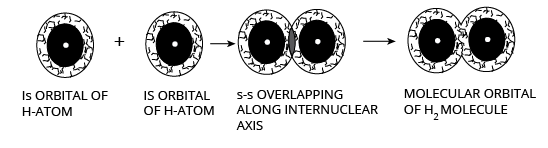Structure of s-s Overlapping

(ii) s-p Overlapping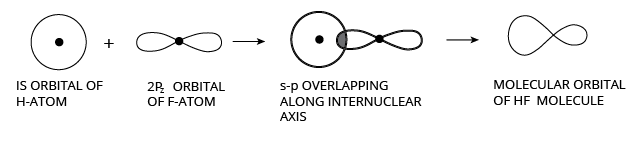Structure of s-p Overlapping

(iii) p-p Overlapping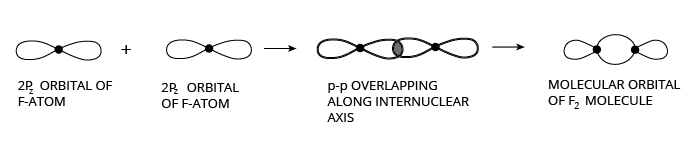Structure of p-p Overlapping

### 2. Pi $\left( \pi \right)$  Bond:

Pi-bonds are formed by lateral (sideways) overlapping of p-orbitals, that is, overlapping of p-orbitals in a direction perpendicular to the internuclear axis (figure).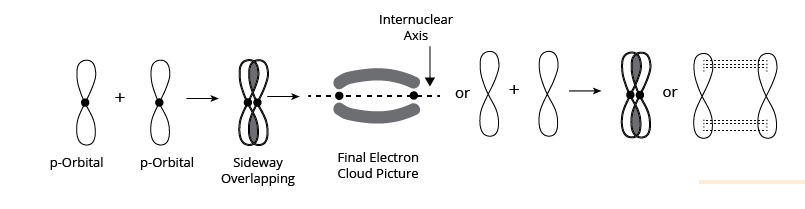Structure of Pi Bond

(i) In the case of an oxygen molecule (each oxygen atom has an electronic configuration of $1{s^2}2{s^2}2{p^2}_x2{p^1}_y2{p^1}_z$  the two atoms are held together by one -bond and one -bond, as shown in the figure.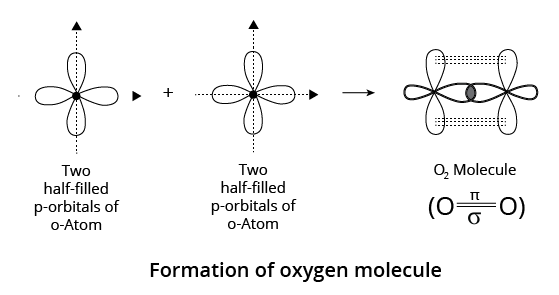p-p Overlapping in Oxygen Molecule

## Bond Parameters

Bond Length:

The bond length is defined as the equilibrium distance between the nuclei of the two bonded atoms.

### Factors Affecting Bond Length

(i) Size of the Atoms:

The bond length rises in proportion to the atom size. Bond lengths of H–X, for example, are in the following order:

HI > HBr > HCl > HF

(ii) Multiplicity of Bond:

The length of the bond decreases as the number of bonds increases. Thus, the bond lengths of carbon-carbon bonds are as follows:

${\rm{C\;}} \equiv {\rm{C < C = C < C - C}}$

(iii) Type of Hybridisation

Since orbitals are smaller in size, they have a higher s-character, a shorter hybrid orbital, and thus a shorter bond length.

### Bond Energy

Bond dissociation enthalpy, or simply bond enthalpy, is the amount of energy required to break one mole of a specific type of bond in order to separate it into gaseous atoms.

## Factors affecting Bond energy

(i) Size of the Atoms:

The greater the atom size, the greater the bond length, and the lower the bond dissociation enthalpy, i.e., the lower the bond strength.

(ii) Multiplicity of Bonds:

This is due to two factors: first, atoms become closer together, and second, the number of bonds that must be broken increases. Bond dissociation enthalpies of H2, O2 and N2, for example, are in the order:

${\rm{H-H < O = O < N\;}} \equiv {\rm{N}}$

(iii) Number of Lone Pairs of Electrons Present:

The greater the number of lone pairs of electrons on the bonded atoms, the greater the repulsion between the atoms and, as a result, the lower the bond dissociation enthalpy.

### Bond Angle

The bond angle is the angle formed by the lines representing the directions of the bonds, i.e. the orbitals containing the bonding electrons.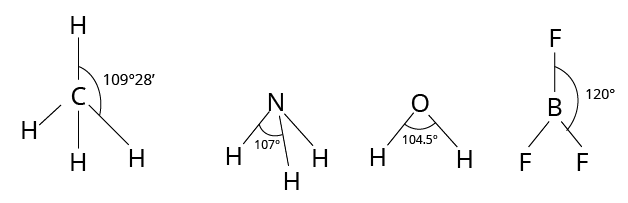Bond Angle

### Bond Order

It is the number of bonds present between two atoms in the Lewis representation of a molecule or ion. The bond orders of a few molecules, for example, are as follows:

 Molecule ${\rm{H}} - {\rm{H}}$ ${\rm{O}} = {\rm{O}}$ ${\rm{N}} \equiv {\rm{N}}$ ${\rm{C}} \equiv {\rm{O}}$ Bond order 1 2 3 3

Bond order can be fractional for odd electron molecules because the three-electron bond is considered equivalent to a half covalent bond. The Lewis structure of NO, for example, is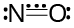Structure of Nitronium Ion

### Molecular Orbital Theory

Another approach to chemical bonding is known as molecular orbital theory (MOT), which was developed by Mulliken (1932) and Hund to better explain bonding characteristics. The molecular orbital theory regards the entire molecule as a unit, with all electrons moving under the influence of all nuclei present. This method recognizes that each electron belongs to the molecule as a whole and can move within it.

### Molecular Orbitals

When the atoms to be bonded come close together, their orbitals lose their individual character and fuse (overlap) to form larger orbitals known as molecular orbitals. A molecule contains molecular orbitals, which are similar to atomic orbitals. The only difference is that electrons in atomic orbitals move under the influence of only one nucleus (i.e., atomic orbitals are monocentric), whereas electrons in molecular orbitals move under the influence of many nuclei (i.e., polycentric).

### Important features of M.O.T.

1. Similar to atomic orbitals, which revolve around an atom's nucleus, molecular orbitals revolve around the nuclei of molecules.

2. Molecular orbitals are not the same as the atomic orbitals from which they are formed.

3. The valence electrons of the constituent atoms are thought to be moving in the molecular orbital under the influence of the nuclei of the participating atoms.

4. Like atomic orbitals in an isolated atom, molecular orbitals have different energy levels.

### Conditions for Atomic Orbitals to Form M.O.:

(i) The combining A.O. must have comparable energy.

(ii) The combining atomic orbitals must overlap to a significant extent; the greater the overlap, the more stable the molecule formed.

### Relative Energies of M.O. and Filling of the Electron:

Energy diagram is shown below: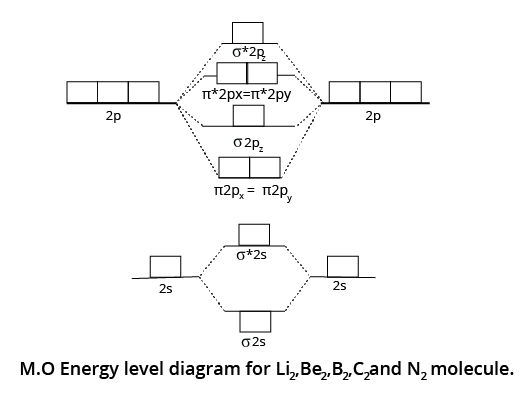Energy Level Diagram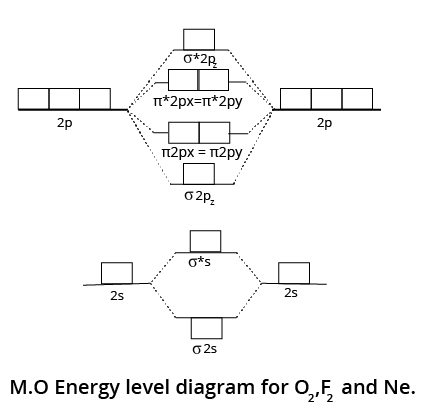Energy Level Diagram

### Solved Examples:

1. Hydrogen bonds are formed in many compounds e.g.,${{\rm{H}}_{\rm{2}}}{\rm{O}},{\rm{HF}},{\rm{N}}{{\rm{H}}_{\rm{3}}}$. The boiling point of such compounds depends to a large extent on the strength of hydrogen bonds and the number of hydrogen bonds. The correct decreasing order of the boiling points of above compounds is:

(a) ${\rm{HF}} > {{\rm{H}}_{\rm{2}}}{\rm{O}} > {\rm{N}}{{\rm{H}}_{\rm{3}}}$

(b) ${{\rm{H}}_{\rm{2}}}{\rm{O}} > {\rm{HF}} > {\rm{N}}{{\rm{H}}_{\rm{3}}}$

(c)${\rm{N}}{{\rm{H}}_{\rm{3}}} > {\rm{HF}} > {{\rm{H}}_{\rm{2}}}{\rm{O}}$

(d) ${\rm{N}}{{\rm{H}}_3} > {{\rm{H}}_{\rm{2}}}{\rm{O}} > {\rm{HF}}$

Correct Option: (b)

Explanation:

The size and the electronegativity are the main factors on which the strength of a compound depends on. As the size of the atom decreases, the electronegativity increases and thus the hydrogen-bonding becomes stronger. Thus, the strength of hydrogen-bonding in the given compounds is:

${{\rm{H}}_{\rm{2}}}{\rm{O}} > {\rm{HF}} > {\rm{N}}{{\rm{H}}_{\rm{3}}}$

2. Which one among the following does not wet the walls of the glass vessel in which it is kept?

(a) Water

(b) Alcohol

(c) Mercury

(d) Phenol

Correct Option: (c)

Explanation: Mercury does not wet the surface of the glass. This is due to the fact that the cohesive forces within the drops are stronger than the adhesive forces between the drops and the glass.

## Importance of Chemical Bonding and Molecular Structure

The study of elements and compounds forms the base of Chemistry. This fundamental chapter holds immense importance in the Class 11 Chemistry syllabus for NEET preparation as it prepares a strong conceptual base to study other chapters in organic and inorganic chemistry.

In this chapter, students will learn about the different chemical bonds and the octet rule of electronic configuration. They will realize how chemical bonds form based on the chemical features of two elements, similar or different.

This chapter will also explain how ions are formed to gain a stable electronic configuration. Every element displays a set of unique features that decides the type of chemical bonding. All types of chemical bonds will be explained elaborately with examples so that students can easily identify the type of compounds.

They will learn the different factors that influence the formation of ionic, covalent, and metallic bonds. They will find out which elements form such bonds and how they can be identified in a compound.

The relevance of the modern periodic table will also be found in the concepts taught in this chapter. You can clearly understand how important this chapter is for completing the entire Chemistry syllabus when you go through the sample papers and previous years’ question papers of NEET.

## Benefits of Chemical Bonding and Molecular Structure Notes

• Considering the importance of this fundamental chapter, the subject matter experts have simplified all the fundamental principles, concepts, derivations, and definitions related to chemical bonding and molecular structure.

• The concise explanation of these concepts will enable you to comprehend the scientific principles behind chemical bonds.

• These revision notes will help you revise it faster before the exam. With the help of Chemical Bonding and Molecular Structure NEET notes, you can easily revise this chapter in no time, thereby saving time to revise the vast NEET Chemistry syllabus before the exam. The quick recall of the formulas and concepts will help you answer faster and more accurately. Thus your NEET score will automatically increase.

## Download Class 11 Chemistry Chemical Bonding and Molecular Structure Notes PDF

You can download the Chemical Bonding and Molecular Structure NEET notes PDF for free from Vedantu and refer to them at your convenience. The experts have already prepared the notes for you, thus you can save the time needed for making notes. Use these revision notes and make this chapter on chemical bonding your strength to score well in the NEET exam.

### Other Important Links Related to NEET Chemical Bonding and Molecular Structure

 Other Important Links for NEET Chemical Bonding and Molecular Structure NEET Chemical Bonding and Molecular Structure Chapter NEET Chemical Bonding and Molecular Structure Important Questions

## FAQs on NEET Revision Notes for Chemistry Chemical Bonding and Molecular Structure

1. What is a chemical bond?

A bond that binds two atoms of the same or different elements is called a chemical bond. It forms when two atoms share or exchange electrons with each other.

2. What is a metallic bond?

The bonds formed in crystal lattices between metals due to the availability of valence electrons are called metallic bonds.

3. What is an ion?

A charged particle formed by an atom by losing or gaining an electron is called an ion. The charge can be positive or negative.

4. Why an atom is neutral?

The balance between the positive charges of protons in the nucleus and the negative charges of electrons in the shells makes an atom neutral.